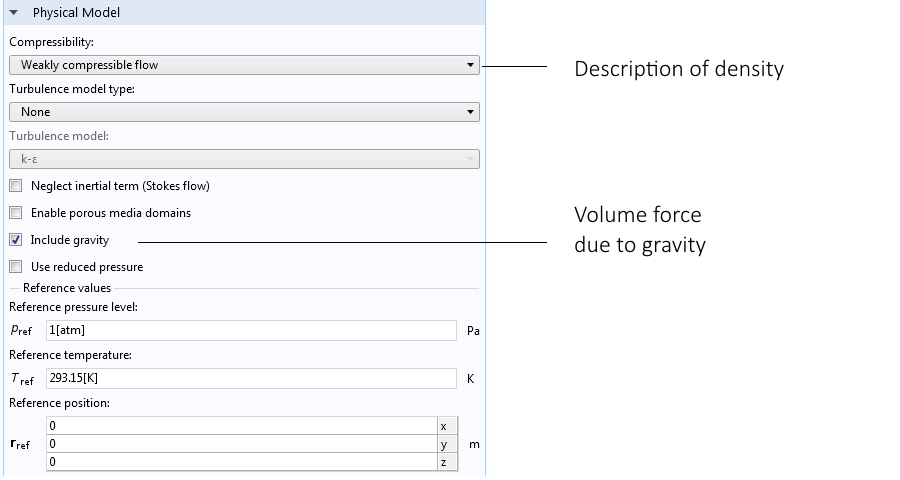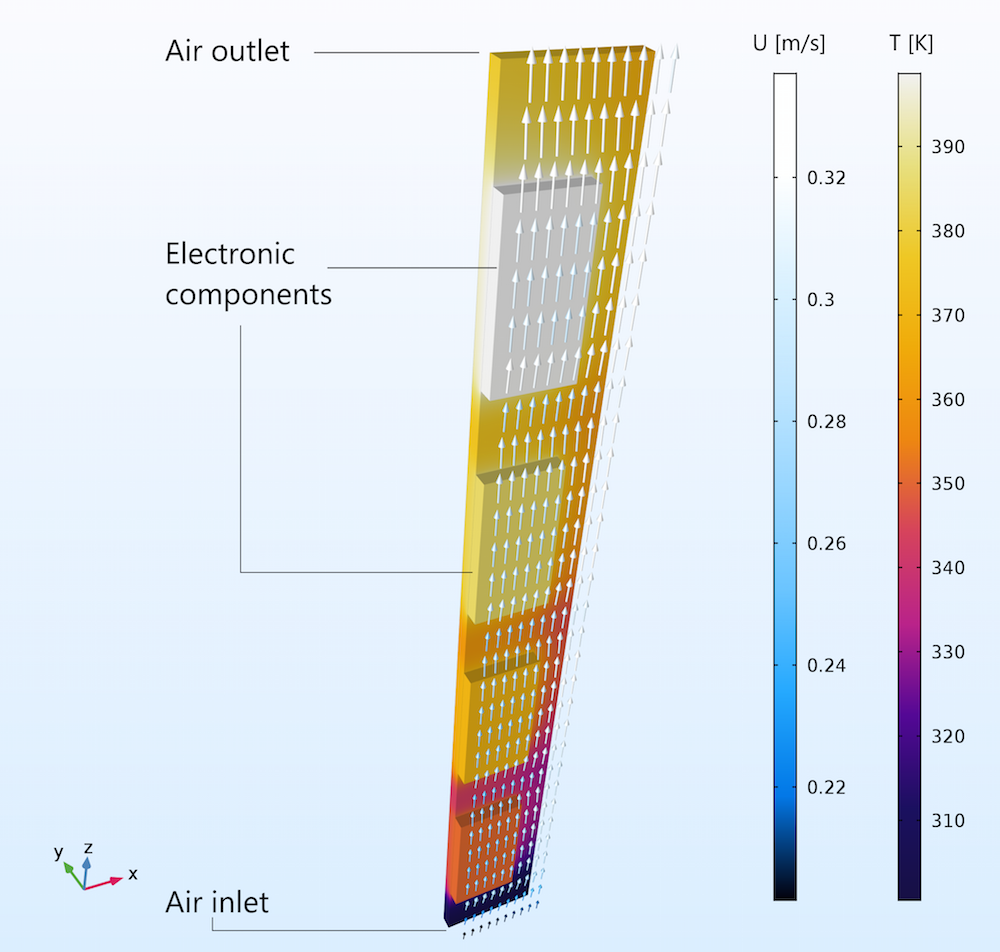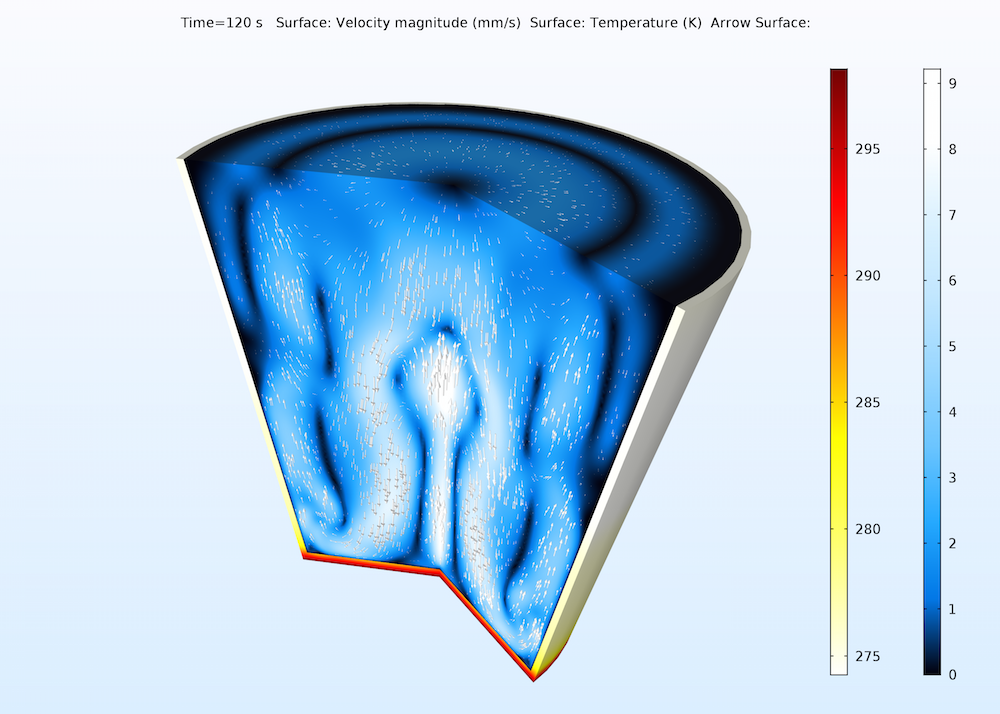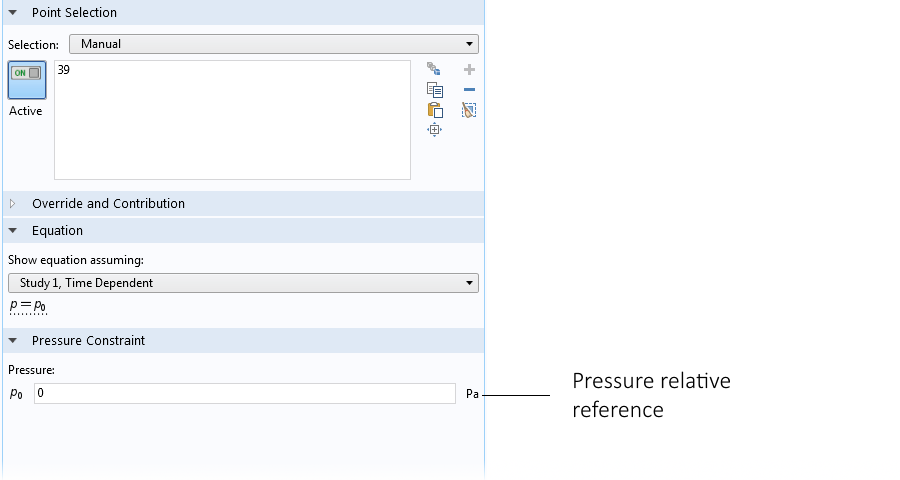# COMSOL Multiphysics® 自然对流建模简介

2016年 12月 23日

### 利用弱可压缩流动选型求解自然对流问题

(1)

$\frac{{\partial \rho }}{{\partial t}} + \nabla \cdot \left( {\rho {\mathbf{u}}} \right) = 0$

(2)

$\frac{{\partial \rho }}{{\partial t}} = \frac{{\partial p}}{{\partial t}}\frac{M}{{RT}} – \frac{{\partial T}}{{\partial t}}\frac{{pM}}{{R{T^2}}}$

(3)

$\frac{{\partial \rho }}{{\partial t}} = – \frac{{\partial T}}{{\partial t}}\frac{{pM}}{{R{T^2}}}$

(4)

$\frac{{\partial T}}{{\partial t}} + \nabla T \cdot {\mathbf{u}} – T\nabla \cdot {\mathbf{u}} = 0$

(5)

${F_z} = -g\rho \left( T \right)$### 使用 Boussinesq 近似的不可压缩流动选项

(6)

$\nabla \cdot {\mathbf{u}} = 0$

(7)

${F_z} = g{\rho _{{\text{ref}}}}\alpha \Delta T$### 约束自然对流模型中的压力方程### 利用耦合或解耦策略求解自然对流问题### 扩展阅读

• 浏览“COMSOL 博客”，了解更多关于自然对流和流体流动建模的知识：

#### 评论 (6)

##### 留言##### 一 王
2017-09-24##### Jialong Wang
2017-10-02

Can I ask how to get Eq.4？I mean the second term in Eq.42017-10-02

Dear Jialong Wang,

Equation 4 is just an expansion of equation 1 using equation 3 and the ideal gas relation rho=pM/RT. So the last term is what remains of the term rho*div(u) after the equation has been divided by the factor pM/RT.

Best regards
COMSOL##### Jialong Wang
2017-10-02

But I got an extra term of -T*grad(p)/p*u##### 钢 罗
2018-06-12##### Tengyue Gao
2018-10-19

Email: support@comsol.com